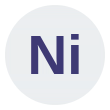# Visualising Solid Shapes

This is an MCQ-based on Visualising Solid Shapes.

This includes cylinder, prism, cube, cone, square pyramid, triangular pyramid, sphere, polyhedrons, polygons, and cuboid.

Start Quiz

What is the difference between a rectangle and a cube?

A rectangle is 3-dimensional and a cube is 2-dimensional. A rectangle and a cube are both 2-dimensional. A rectangle is 2-dimensional and a cube is 3-dimensional. A rectangle and a cube are both 3-dimensional.

The top-view of a cube looks like a:

Circle Square Rectangle Triangle

How many circular bases does a cylinder have?

2 3 4 1

How many faces does a cube have ?

6 4 3 2.

How many vertices does a cuboid have ?

4 6 8 3.

For a polyhedron, if ‘F’ stands for number of faces, V stands for number of vertices and E stands for number of edges, then which of the following relationships is named as Euler’s formula ?

F + V = E + 2 F + E = V + 2 V + E = F + 2 F+ V = E – 2.

The side-view of a cone appears as:

Circle Square Rectangle Triangle

How many vertices does a triangular pyramid have ?

1 2 3 4

If a polyhedron has 6 vertices and 12 edges. What is the number of faces it has ?

6 8 12 18

A sphere has how many faces ?

One Two Four None

How many vertices does a pyramid with square base have ?

5 4 3 6

How many vertices does a triangular prism have ?

6 5 4 3

How many edges does a cube have ?

12 6 4 8.

Prisms and Pyramids are:

Polygons Cubes Polyhedrons Spherical shapes

Number of vertices a cone has:

One Two Three Four

An example of cone is:

Tube A tent A ball A box

The top-view of a cuboid looks like a:

Circle Square Rectangle Triangle

A three dimensional shape is _________ object.

Solid

2d

Plane

None of these

The example of cylindrical shape is:

Pipes

Gas cylinder

Cold drink cans

All of the above

Quiz/Test Summary
Title: Visualising Solid Shapes
Questions: 19
Contributed by: